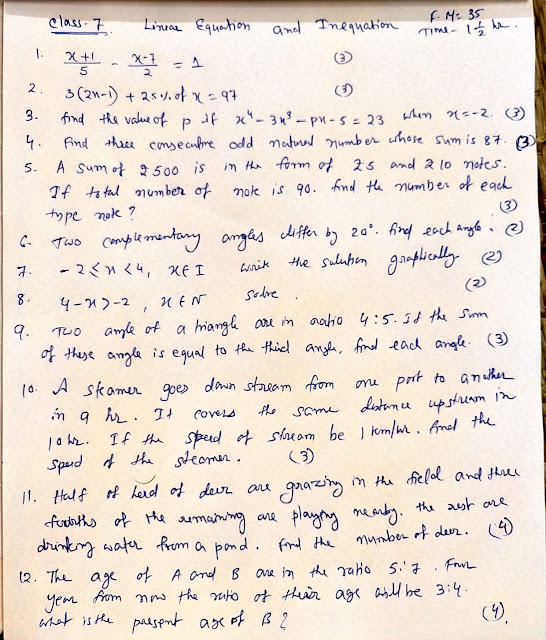## 6 Dec 2020

### Two equal circles passes through the centres of each other and intersect each other at A and B. A line passes through point A intersecting the two circles at points C and D. How can I prove that the triangle formed by the points B,C and D is equilateral?

Join AB and Center of two circle X Y.

Now, AX= AY=XY ( as circle are equal and radius of same circle)

and, XB=BY=XY

so Triangle AXY is a Equilateral Triangle, so each angle = 60 degree

similarly, triangle BXY is a Equilateral Triangle, so each angle = 60 degree

So, <AXY= 120 degree and < AYB = 120 degree

now, <ACB=1/2 <AXY= 60 degree

and <ADB= 1/2 < AYB= 60 degree

so, < CBD=180-60-60=60 degree

as all angle of triangle BCD is 60 degree than BCD is a equilateral triangle.